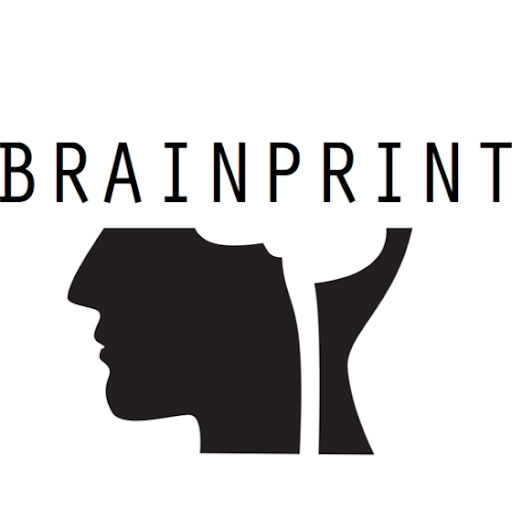RgrNormand -4 years ago 111
R Question

# Plotly - Surface - Text hoverinfo not working

I have build a surface chart with plotly and I am trying to have hoverinfo based on my own text. Curiously it is not working anymore.

``````library(plotly)
x <- rnorm(10)
y <- rnorm(10)
z <- outer(y, x)

p <- plot_ly(x = ~x, y = ~y, z = ~z, type = "surface",
text = ~paste0("My X = ", x, "\n My Y = ", y, "\n My Z = ", z),
hoverinfo = "text") %>% layout(dragmode = "turntable")
print(p)
``````

Although

``````p <- plot_ly(x = ~x, y = ~y, z = ~z, type = "surface") %>% layout(dragmode = "turntable")
``````

works well.

I have also tried to substitute
`\n`
by
`<br />`
with no effect.

I am using R 3.4.0 and plotly 4.7.0 on macOS Sierra.

Any suggestions?Nate Day
Answer Source

Plotly's labeling seems finicky with custom labels using the `~paste()` syntax because it is trying to build a new data structure with your inputs (three vectors and one matrix), but if you pass in custom labels as a `matrix` with the same dimensions it will work.

``````custom_txt <- paste0("My X = ", rep(x, times = 10),
"</br> My Y = ", rep(y, each = 10), # correct break syntax
"</br> My Z = ", z) %>%
matrix(10,10) # dim must match plotly's under-the-hood? matrix

plot_ly(x = ~x, y = ~y, z = ~z, type = "surface",
text = custom_txt,
hoverinfo = "text") %>%
layout(dragmode = "turntable")
``````
Recommended from our users: Dynamic Network Monitoring from WhatsUp Gold from IPSwitch. Free Download
Latest added